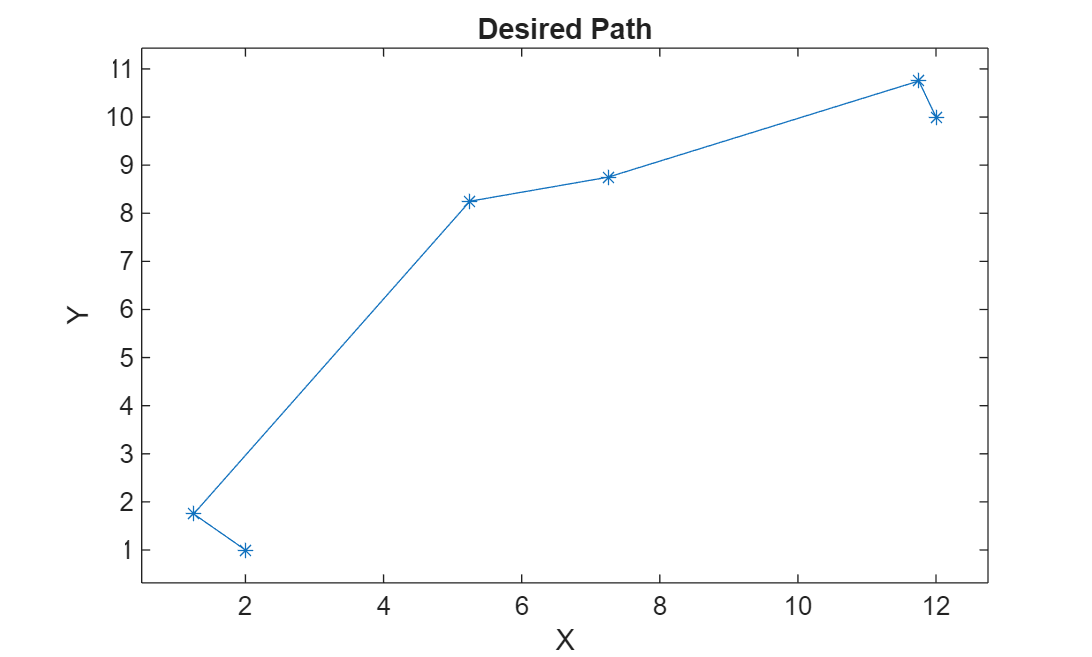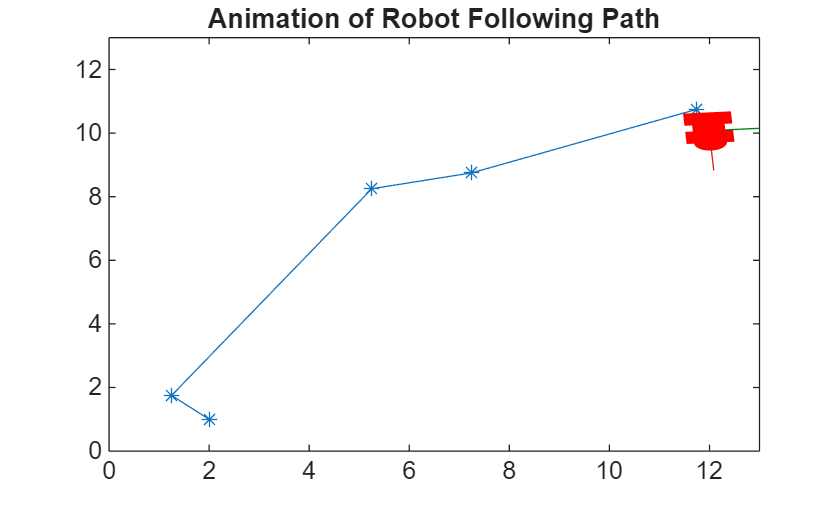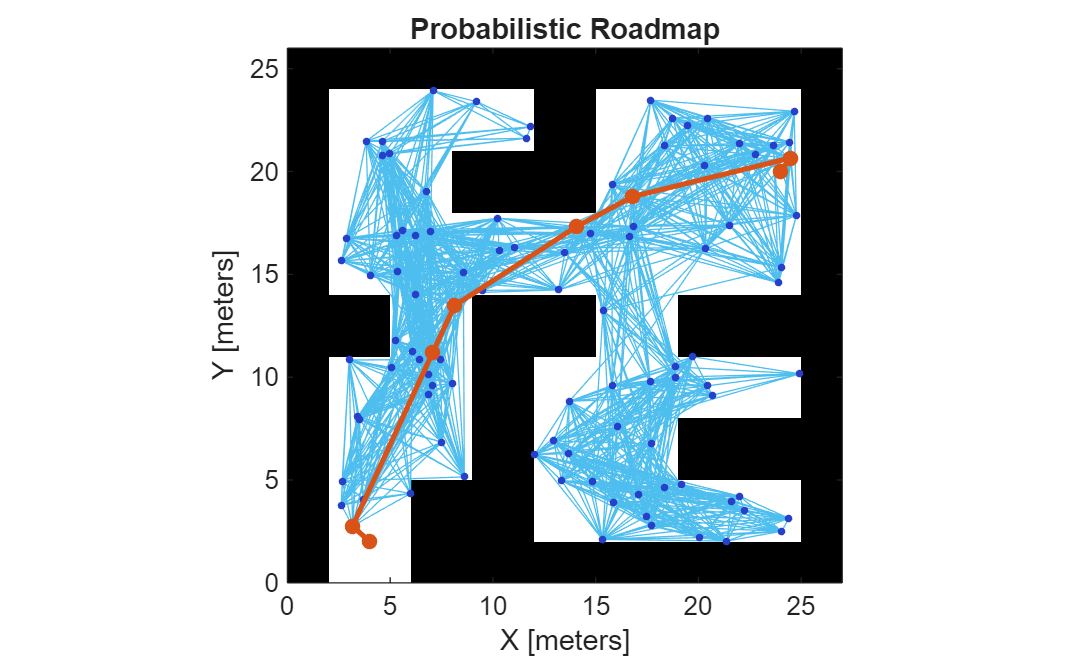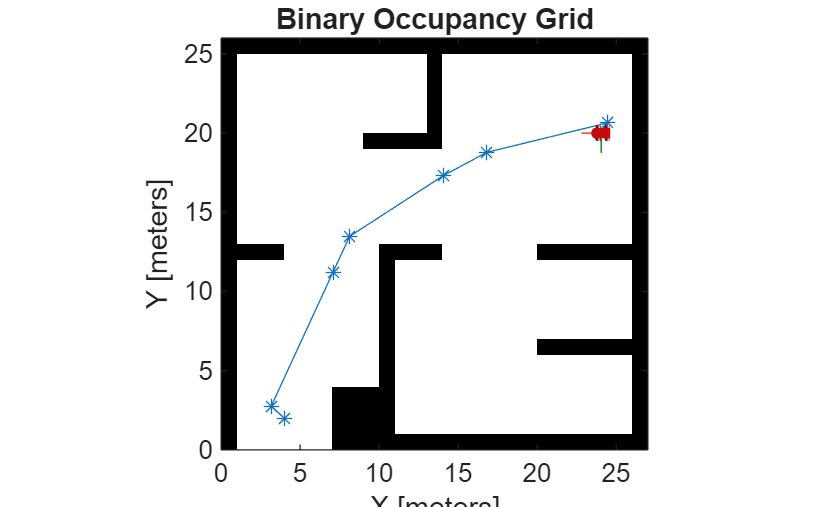Documentation

### This is machine translation

Mouseover text to see original. Click the button below to return to the English version of the page.

Note: This page has been translated by MathWorks. Click here to see
To view all translated materials including this page, select Country from the country navigator on the bottom of this page.

## Path Following for a Differential Drive Robot

This example demonstrates how to control a robot to follow a desired path using a Robot Simulator. The example uses the Pure Pursuit path following controller to drive a simulated robot along a predetermined path. A desired path is a set of waypoints defined explicitly or computed using a path planner (refer to Path Planning in Environments of Different Complexity). The Pure Pursuit path following controller for a simulated differential drive robot is created and computes the control commands to follow a given path. The computed control commands are used to drive the simulated robot along the desired trajectory to follow the desired path based on the Pure Pursuit controller.

Note: Starting in R2016b, instead of using the step method to perform the operation defined by the System object™, you can call the object with arguments, as if it were a function. For example, `y = step(obj,x)` and `y = obj(x)` perform equivalent operations.

### Define Waypoints

Define a set of waypoints for the desired path for the robot

```path = [2.00 1.00; 1.25 1.75; 5.25 8.25; 7.25 8.75; 11.75 10.75; 12.00 10.00]; ```

Set the current location and the goal location of the robot as defined by the path.

```robotCurrentLocation = path(1,:); robotGoal = path(end,:);```

Assume an initial robot orientation (the robot orientation is the angle between the robot heading and the positive X-axis, measured counterclockwise).

`initialOrientation = 0;`

Define the current pose for the robot [x y theta]

`robotCurrentPose = [robotCurrentLocation initialOrientation];`

### Initialize the Robot Simulator

A simple robot simulator is used in this example that updates and returns the pose of the differential drive robot for given control inputs. An external simulator or a physical robot will require a localization mechanism to provide an updated pose of the robot.

Initialize the robot simulator and assign an initial pose. The simulated robot has kinematic equations for the motion of a two-wheeled differential drive robot. The inputs to this simulated robot are linear and angular velocities. It also has plotting capabilities to display the robot's current location and draw the trajectory of the robot.

```robotRadius = 0.4; robot = ExampleHelperRobotSimulator('emptyMap',2); robot.enableLaser(false); robot.setRobotSize(robotRadius); robot.showTrajectory(true); robot.setRobotPose(robotCurrentPose);```

Visualize the desired path

```plot(path(:,1), path(:,2),'k--d') xlim([0 13]) ylim([0 13])```### Define the Path Following Controller

Based on the path defined above and a robot motion model, you need a path following controller to drive the robot along the path. Create the path following controller using the `robotics.PurePursuit` object.

`controller = robotics.PurePursuit;`

Use the path defined above to set the desired waypoints for the controller

`controller.Waypoints = path;`

Set the path following controller parameters. The desired linear velocity is set to 0.3 meters/second for this example.

`controller.DesiredLinearVelocity = 0.3;`

The maximum angular velocity acts as a saturation limit for rotational velocity, which is set at 2 radians/second for this example.

`controller.MaxAngularVelocity = 2;`

As a general rule, the lookahead distance should be larger than the desired linear velocity for a smooth path. The robot might cut corners when the lookahead distance is large. In contrast, a small lookahead distance can result in an unstable path following behavior. A value of 0.5 m was chosen for this example.

`controller.LookaheadDistance = 0.5;`

### Using the Path Following Controller, Drive the Robot over the Desired Waypoints

The path following controller provides input control signals for the robot, which the robot uses to drive itself along the desired path.

Define a goal radius, which is the desired distance threshold between the robot's final location and the goal location. Once the robot is within this distance from the goal, it will stop. Also, you compute the current distance between the robot location and the goal location. This distance is continuously checked against the goal radius and the robot stops when this distance is less than the goal radius.

Note that too small value of the goal radius may cause the robot to miss the goal, which may result in an unexpected behavior near the goal.

```goalRadius = 0.1; distanceToGoal = norm(robotCurrentLocation - robotGoal);```

The `robotics.PurePursuit` object computes control commands for the robot. Drive the robot using these control commands until it reaches within the goal radius. If you are using an external simulator or a physical robot, then the controller outputs should be applied to the robot and a localization system may be required to update the pose of the robot. The controller runs at 10 Hz.

```controlRate = robotics.Rate(10); while( distanceToGoal > goalRadius ) % Compute the controller outputs, i.e., the inputs to the robot [v, omega] = controller(robot.getRobotPose); % Simulate the robot using the controller outputs. drive(robot, v, omega); % Extract current location information ([X,Y]) from the current pose of the % robot robotCurrentPose = robot.getRobotPose; % Re-compute the distance to the goal distanceToGoal = norm(robotCurrentPose(1:2) - robotGoal); waitfor(controlRate); end```The simulated robot has reached the goal location using the path following controller along the desired path. Close simulation.

`delete(robot)`

### Using the Path Following Controller Along with PRM

If the desired set of waypoints are computed by a path planner, the path following controller can be used in the same fashion. Start the robot simulator with a simple map.

```robot = ExampleHelperRobotSimulator('simpleMap',2); robot.enableLaser(false); robot.setRobotSize(robotRadius);````robot.showTrajectory(true);`

You can compute the `path` using the PRM path planning algorithm. See Path Planning in Environments of Different Complexity for details.

```mapInflated = copy(robot.Map); inflate(mapInflated,robotRadius); prm = robotics.PRM(mapInflated); prm.NumNodes = 100; prm.ConnectionDistance = 10;```

Find a path between the start and end location. Note that the `path` will be different due to the probabilistic nature of the PRM algorithm.

```startLocation = [2.0 1.0]; endLocation = [12.0 10.0]; path = findpath(prm, startLocation, endLocation)```
```path = 6×2 2.0000 1.0000 1.5851 1.3808 4.0668 6.7417 7.0353 8.6624 11.8252 10.6359 12.0000 10.0000 ```

Display the path

`show(prm, 'Map', 'off', 'Roadmap', 'off');`You defined a path following controller above which you can re-use for computing the control commands of a robot on this map. To re-use the controller and redefine the waypoints while keeping the other information the same, use the `release` function.

```release(controller); controller.Waypoints = path;```

Set current location and the goal of the robot as defined by the path

```robotCurrentLocation = path(1,:); robotGoal = path(end,:);```

Assume an initial robot orientation

`initialOrientation = 0;`

Define the current pose for robot motion [x y theta]

`robotCurrentPose = [robotCurrentLocation initialOrientation];`

Reset the current position of the simulated robot to the start of the path.

```robot.setRobotPose(robotCurrentPose) show(prm, 'Map', 'off', 'Roadmap', 'off');```Compute distance to the goal location

`distanceToGoal = norm(robotCurrentLocation - robotGoal);`

Define a goal radius

`goalRadius = 0.1;`

Drive the robot using the controller output on the given map until it reaches the goal. The controller runs at 10 Hz.

```reset(controlRate); while( distanceToGoal > goalRadius ) % Compute the controller outputs, i.e., the inputs to the robot [v, omega] = controller(robot.getRobotPose); % Simulate the robot using the controller outputs drive(robot, v, omega); % Extract current location information from the current pose robotCurrentPose = robot.getRobotPose; % Re-compute the distance to the goal distanceToGoal = norm(robotCurrentPose(1:2) - robotGoal); waitfor(controlRate); end```The simulated robot has reached the goal location using the path following controller along the desired path. Stop the robot.

`drive(robot, 0, 0);`

Close Simulation.

`delete(robot);`

### See Also

##### SupportGet trial now